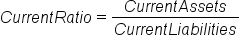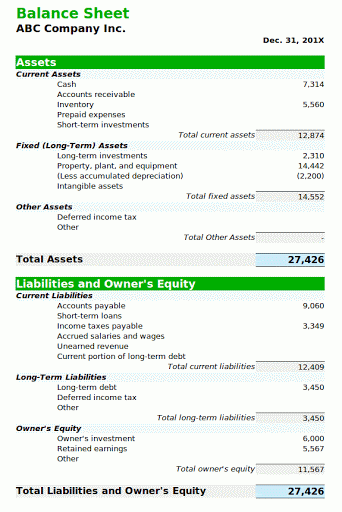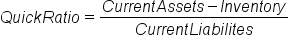+

# Liquidity Ratios

Author: Sophia Tutorial
##### Description:

Interpret or calculate the ratios that measure short term financial strength.

(more)### Developing Effective Teams

*No strings attached. This college course is 100% free and is worth 1 semester credit.

37 Sophia partners guarantee credit transfer.

299 Institutions have accepted or given pre-approval for credit transfer.

* The American Council on Education's College Credit Recommendation Service (ACE Credit®) has evaluated and recommended college credit for 32 of Sophia’s online courses. Many different colleges and universities consider ACE CREDIT recommendations in determining the applicability to their course and degree programs.

Tutorial

what's covered
In this lesson, you will learn about ratios that measure short-term financial strength. Specifically, this lesson will cover:
1. Current Ratio
2. Quick Ratio

## 1. Current Ratio

Recall that the concept of liquidity represents the ability of a company to repay its short-term creditors out of the cash that it has available. This liquidity can be represented by a ratio that compares assets to liabilities. There are a couple of ways to do this:

• Current ratio
• Quick ratio
The current ratio measures whether or not a firm has enough resources to pay its debts over the next 12 months. It compares the current assets to the current liabilities. These current assets comprise assets that can be converted to cash or used to pay current liabilities within the coming year. Current assets usually include cash, cash equivalents, short-term investments, accounts receivable, and inventory. Current liabilities are often represented by all of the liabilities that an organization has to be settled in cash within the next year.

The current ratio is found by dividing the current assets by the current liabilities.

formula

Current RatioAcceptable current ratios vary from industry to industry but they are generally between one and a half and three for a healthy business. If a company has a ratio in this range, it generally indicates good short-term financial strength.

EXAMPLE

Here is the balance sheet of our fictitious ABC Company.The total current assets are \$12,874, while the total current liabilities are \$12,409. If we divide the current assets by the current liabilities, we get 1.05, which seems a little bit low. The company can barely repay its current liabilities from its current assets.

Now, can a current ratio be too high? If you are a shareholder and the current ratio is 4, are you happy because they are so liquid? Most likely, you would want that number to be lower, because that higher number means there is cash sitting there that is not being invested in growth or paid out in dividends.

term to know

Current Ratio
A ratio that measures whether or not a firm has enough resources to pay its debts over the next 12 months.

## 2. Quick Ratio

The quick ratio, or the acid test, measures the ability of a company to use its cash or quick assets to retire its liabilities immediately. It is calculated by dividing the current assets minus the inventory by the current liabilities.

formula

Quick RatioCash and cash equivalents are the most liquid assets found in the current asset portion of the balance sheet and among the ones that could be most quickly converted; we can include accounts receivable among those as well. However, inventory is something that could take a bit longer to convert, so it would not be immediately available to repay the current liabilities. Because of this, inventory is excluded in the quick ratio formula.

EXAMPLE

Let's take a look at the balance sheet for ABC Company again.We said that ABC Company has current assets of \$12,874, but if we subtract the inventory of \$5,560, then we only have the cash of \$7,314. If we divide that by the current liabilities of \$12,409, then we arrive at a quick ratio of 0.65. This shows we do not have nearly enough coverage to take care of our current liabilities in the short term.

term to know

Quick Ratio
A ratio that measures the ability of a company to use its cash or quick assets to retire its liabilities immediately.
summary
In this lesson, you learned that liquidity is the ability of an organization to pay its current liabilities with its current assets. We can measure this with the current ratio, which is current assets divided by current liabilities. Generally, this should be between 1.5 and 3 for healthy businesses. The second measure that you looked at was the quick ratio, which subtracts the inventory from the current assets. The quick ratio should be one or higher, but this can vary.

Best of luck in your learning!

Source: THIS CONTENT HAS BEEN ADAPTED FROM LUMEN LEARNING'S “LIQUIDITY RATIOS” TUTORIAL.

Terms to Know
Current Ratio

A ratio that measures whether or not a firm has enough resources to pay its debts over the next 12 months.

Quick Ratio

A ratio that measures the ability of a company to use its cash or quick assets to retire its liabilities immediately.

Formulas to Know
Current RatioQuick RatioRating